# Basic Geometry : How to find the area of a circle

## Example Questions

← Previous 1 3 4 5 6 7 8 9 15 16

### Example Question #1 : How To Find The Area Of A Circle

The radius of a circle is 4 cm, what is the area?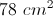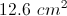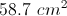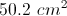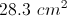Explanation:

The area of a circle is found by: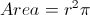, where r is the radius.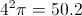.

The area of the circle is.

### Example Question #2 : How To Find The Area Of A Circle

The radius,, of the circle below is 18 units. What is the area of the circle?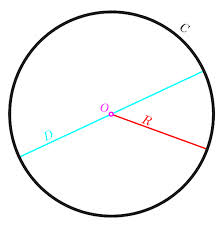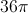square units

Cannot be determined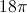square units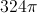square units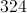square unitssquare units

Explanation:

The formula for the area,, of a circle with radiusis: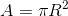We can fill in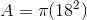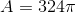You could do the arithmetic to get an area of about 1,017.876 square units, but it is ok and more precise to leave it as shown.

### Example Question #1 : Radius

Give the area of a circle with diameter 13.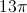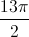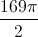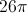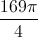Explanation:

Half of the diameter 13 is the radius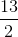. Use the area formula: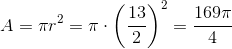### Example Question #1 : Radius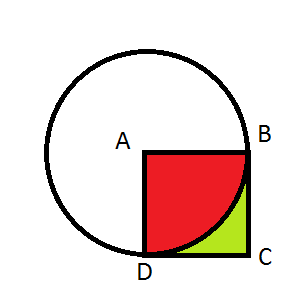Point A is the center of the circle above.

Figure ABCD is a square.

The radius of the circle isunits.

Find the area of the red-colored shape.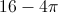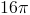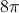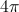Explanation:

Since it is stated that A is the center of the circle and ABCD is a square, angle BAD is a right angle that comprises exactly one fourth of the circle (90o is one fourth of 360o). This can also be seen through a proportion.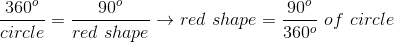Since the angle that contains the red area is one fourth of the circle, finding the area of the entire circle, and then one fourth of that number gives the answer. Use the equation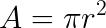to find the area of a circle, whereis area andis the radius.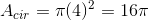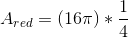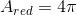### Example Question #5 : How To Find The Area Of A Circle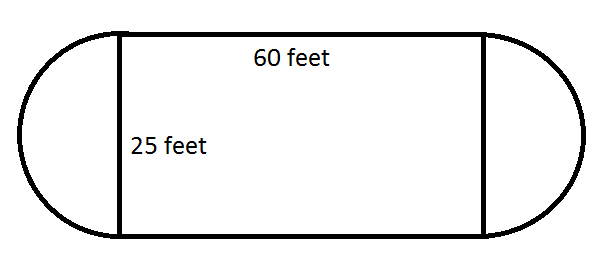Find the area of the ice rink formed by the rectangle and semi-circles with the dimensions shown.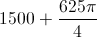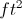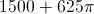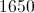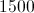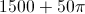Explanation:

To find the area of the entire rink, find the area of the rectangle in the middle of the rink and add it to the area of the circle that would be formed by the two semi-crcles on the edges of that rectangle.

The area of the rectangle is found by multiplying its width by its length.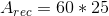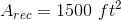To find the area of the circle that accounts for the two semi-circles, use the formula, whereis the radius of the circle.

The radius of the circle is half of its diameter. The diameter of the circle is the same as the width of the rectangle in the case of this rink, and half of that is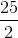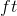.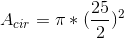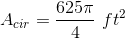Adding this area to the area of the rectangle gives the area of the entire rink.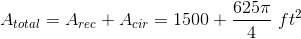### Example Question #1 : How To Find The Area Of A Circle

If a circle has circumference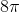, what is its area?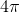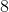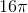Explanation:

If the circumference is, then since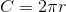we know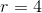. We further know that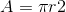, so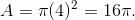### Example Question #61 : Geometry

If the equation of a circle is (x – 7)+ (y + 1)= 81, what is the area of the circle?

81π

18π

6561π

49π

81π

Explanation:

The equation is already in a circle equation, and the right side of the equation stands for r2 → r= 81 and r = 9

The area of a circle is πr2, so the area of this circle is 81π.

### Example Question #8 : How To Find The Area Of A Circle

Assume π = 3.14

A man would like to put a circular whirlpool in his backyard. He would like the whirlpool to be six feet wide. His backyard is 8 feet long by 7 feet wide. By state regulation, in order to put a whirlpool in a backyard space, the space must be 1.5 times bigger than the pool. Can the man legally install the whirlpool?

No, because the area of the backyard is smaller than the area of the whirlpool.

Yes, because the area of the whirlpool is 28.26 square feet and 1.5 times its area would be less than the area of the backyard.

No, because the area of the backyard is 30 square feet and therefore the whirlpool is too big to meet the legal requirement.

No, because the area of the whirlpool is 42.39 square feet and 1.5 times its area would be greater than the area of the backyard.

Yes, because the area of the whirlpool is 18.84 square feet and 1.5 times its area would be less than the area of the backyard.

Yes, because the area of the whirlpool is 28.26 square feet and 1.5 times its area would be less than the area of the backyard.

Explanation:

If you answered that the whirlpool’s area is 18.84 feet and therefore fits, you are incorrect because 18.84 is the circumference of the whirlpool, not the area.

If you answered that the area of the whirlpool is 56.52 feet, you multiplied the area of the whirlpool by 1.5 and assumed that that was the correct area, not the legal limit.

If you answered that the area of the backyard was smaller than the area of the whirlpool, you did not calculate area correctly.

And if you thought the area of the backyard was 30 feet, you found the perimeter of the backyard, not the area.

The correct answer is that the area of the whirlpool is 28.26 feet and, when multiplied by 1.5 = 42.39, which is smaller than the area of the backyard, which is 56 square feet.

### Example Question #1 : Radius

There are two identical circles on a plane that overlap. The radius of both circles is 1. The region in which they overlap has an area of π.

What is the total area of the 2 overlapping circles?

2π

π

1

0

2

π

Explanation:

The total area of both circles is π + π = 2π

Since the region overlaps, we cannot count it twice, so we must subtract it.

we get 2π – π = π

### Example Question #62 : Geometry

A square with a side length of 4 inches is inscribed in a circle, as shown below. What is the area of the unshaded region inside of the circle, in square inches?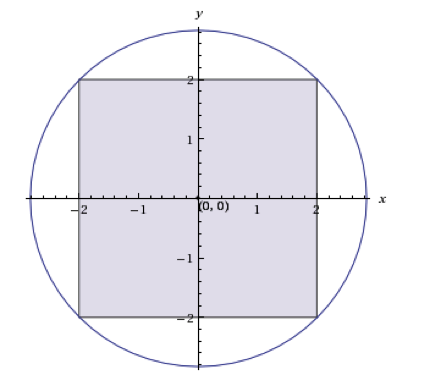8π-4

4π-4

8π - 16

2π-4

8π-8